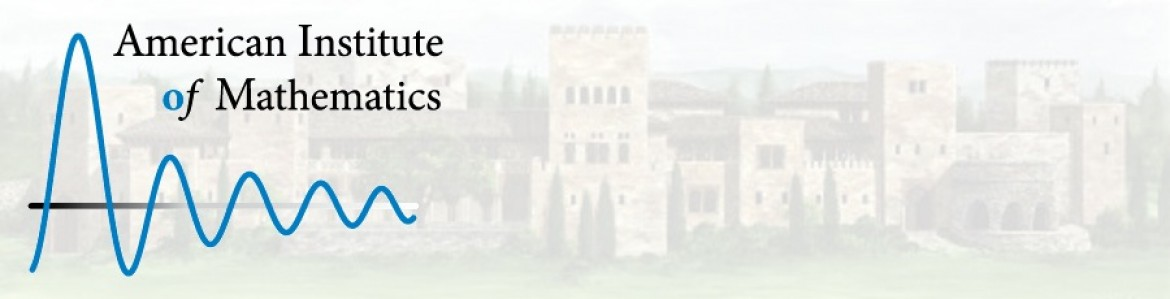Select to expand
Select to collapse

# Course ePortfolio

## Introduction to Linear Algebra

This collection contains the California Open Educational Resources Council (CA OER) list of peer-reviewed textbooks for Linear Algebra (common course-id MATH 250), one of the top 50 college courses identified for inclusion in the California Open Online Library for Education (COOLforEd).

CID Number: MATH 250

General Course Description - MATH 250:

This course develops the techniques and theory needed to solve and classify systems of linear equations. Solution techniques include row operations, Gaussian elimination, and matrix algebra. Investigates the properties of vectors in two and three dimensions, leading to the notion of an abstract vector space. Vector space and matrix theory are presented including topics such as inner products, norms, orthogonality, eigenvalues, eigenspaces, and linear transformations. Selected applications of linear algebra are included.

Minimum units: 3.0

Any rationale or comment: None

Share

### Prerequisites

Prerequisites: Calculus I (C-ID MATH 210, MATH 211 OR MATH 900S)

Corequisites: None

Advisories/Recommendations: A year of college calculus. Prior or concurrent course work with vector calculus or vector-intensive physics would be helpful.

### Pedagogical Approach & Learning Outcomes

None

#### Learning Outcomes

Upon successful completion of the course, students will be able to:

• Find solutions of systems of equations using various methods appropriate to lower division linear algebra;
• Use bases and orthonormal bases to solve problems in linear algebra;
• Find the dimension of spaces such as those associated with matrices and linear transformations;
• Find eigenvalues and eigenvectors and use them in applications; and
• Prove basic results in linear algebra using appropriate proof-writing techniques such as linear independence of vectors; properties of subspaces; linearity, injectivity and surjectivity of functions; and properties of eigenvectors and eigenvalues.

### Assessment & Other Information

#### Assessment

• Tests,
• examinations,
• homework or
• projects

where students demonstrate their mastery of the learning objectives and their ability to devise, organize and present complete solutions to problems and proofs.

None

### Course Resources

•A First Course in Linear Algebra (Open (Access) Textbook)
A First Course in Linear Algebra is an introductory textbook aimed at college-level sophomores and juniors. Typically... More
•Linear Algebra (Open (Access) Textbook)
From the author’s description of the book: The approach is developmental. Although the presentation is focused on... More
•Linear Algebra Done Wrong (Open (Access) Textbook)
Brown University has two introductory linear algebra courses. This text is used in the honors course that emphasizes... More